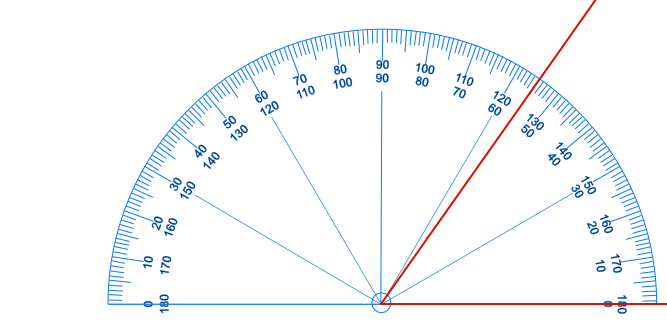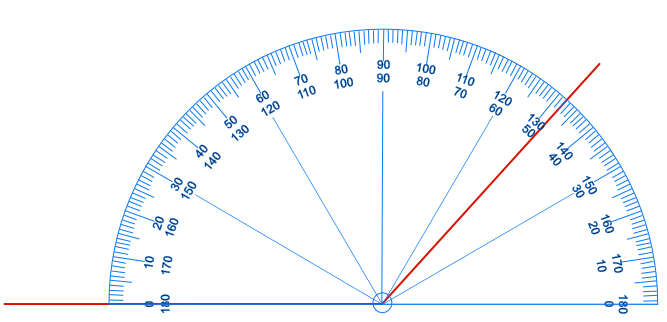Measuring Angles

# Measuring Angles

Basic, GCSE(F),

Angles are measured using a protractor. A protractor measures in degrees. A protractor is normally a semi-circle (as found in geometry sets) or a complete circle.

There are four basic steps to measuring an angle accurately using a protractor.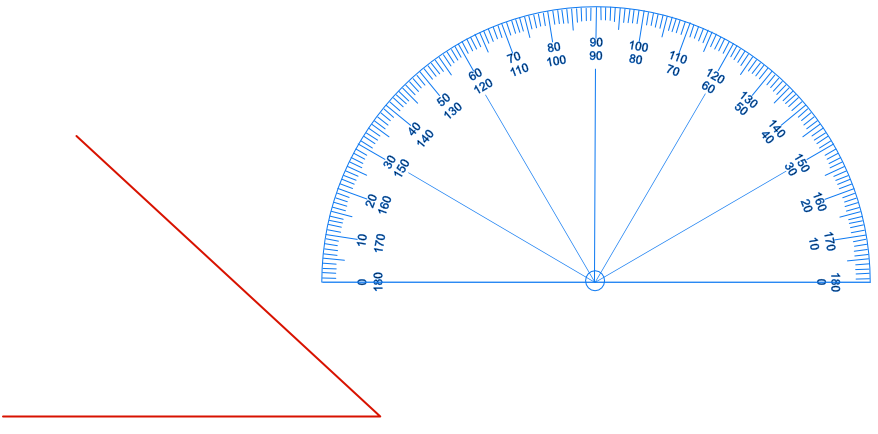1. Place the centre of the protractor (the middle of the bottom line, normally shown with a circle) exactly on the point of the angle being measured: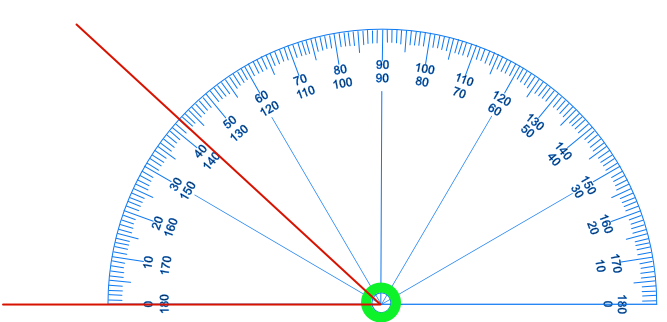2. Make sure that the base line of the protractor is exactly on top of one of the lines of the angle: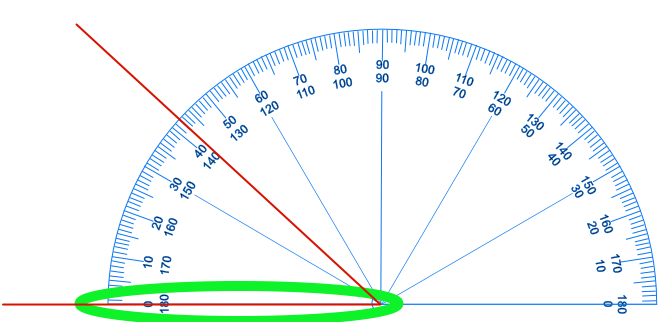3. Check if the inner scale (of numbers) or the outer scale is being used by determining which scale is at 0º on the line: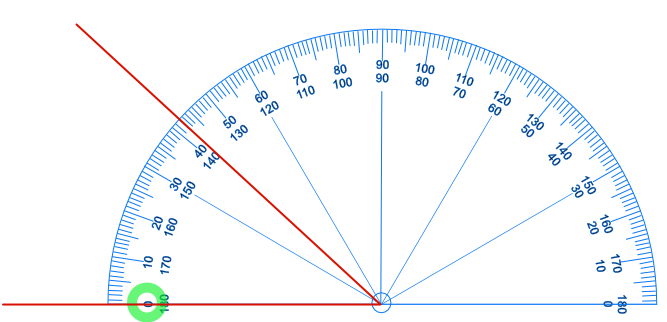4. Read the angle measurement from the correct (inner or outer) scale: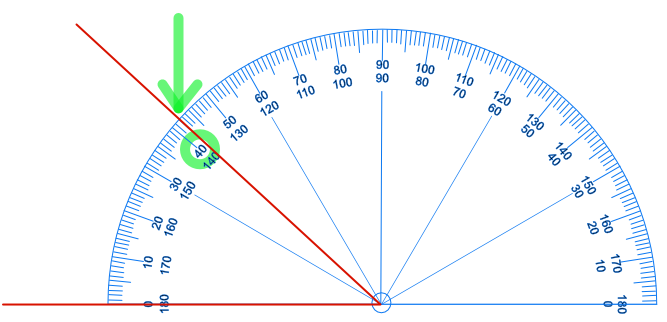In this instance, the angle being measured is 42º

## Examples

1. What is the size of the angle being measured below?